Geometry

Geometry is all about shapes and their properties.

If you like playing with objects, or like drawing, then geometry is for you!

Geometry can be divided into:Plane Geometry is about flat shapes like lines, circles and triangles ... shapes that can be drawn on a piece of paperSolid Geometry is about three dimensional objects like cubes, prisms, cylinders and spheres.Hint: Try drawing some of the shapes and angles as you learn ... it helps.

Point, Line, Plane and SolidA Point has no dimensions, only position
A Line is one-dimensional
A Plane is two dimensional (2D)
A Solid is three-dimensional (3D)

Why?

Why do we do Geometry? To discover patterns, find areas, volumes, lengths and angles, and better understand the world around us.

Plane Geometry

Plane Geometry is all about shapes on a flat surface (like on an endless piece of paper).Polygons

A Polygon is a 2-dimensional shape made of straight lines. Triangles and Rectangles are polygons.

Here are some more:PentagonPentagramHexagonSymbols

There are many special symbols used in Geometry. Here is a short reference for you:

Geometric Symbols

Angles

Types of AnglesAcute Angles Right Angles Obtuse Angles Straight Angle Reflex Angles Full Rotation

Using Drafting ToolsTransformations and SymmetryCoordinatesMore Advanced Topics in Plane Geometry

PythagorasConic Sections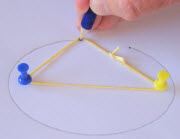Triangle Centers

TrigonometryTrigonometry is a special subject of its own, so you might like to visit:

Solid Geometry

Solid Geometry is the geometry of three-dimensional space - the kind of space we live in ...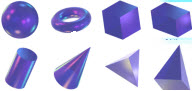Common 3D Shapes

Polyhedra and Non-Polyhedra

There are two main types of solids, "Polyhedra", and "Non-Polyhedra":

Polyhedra (they must have flat faces):

Non-Polyhedra (when any surface is not flat):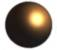SphereTorus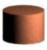Cylinder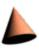Cone